# 3: Variables and Operators

## Learning Goals

At the end of this Lesson you will be able to:

• Declare string and numeric variables and assign values to them with the let keyword.
• Apply the correct variable naming standards
• Perform addition, subtraction, multiplication and division on numeric variables
• Follow the rules of operator precedence in arithmetic statements
• Apply the assignment operator correctly in statements that contain variables and values, and variables only
• Reveal a variable's type using the typeof operator.

In this Lesson you will meet the following terms:

• Variable
• String variable
• Numeric variable
• Assignment statement

### Exercise Files

In this Lesson you will work with the following web pages:

You will find these files in your 📁 /javascript/exercises/3/ sub-folder.

You can think of a variable as a container or ‘storage box’ for a value that may be different each time the JavaScript code is run.

Consider the example of a website login page that asks for a user's First Name.The value entered might be 'Mary' or 'John' or whatever. But in the JavaScript code that stores the value, the variable will always have the same name of, for example, userSurname.

In JavaScript the first thing you do with a variable is declare it. The following code declares the variable named FirstName.

```var FirstName;
```

The declaration begins with the word var. This so-called keyword allocates memory storage space for new data and tells JavaScript that a new variable name is in use.

The more modern version of JavaScript uses the keywords const or let to declare a variable. For simplicity, the let keyword is always used in the examples in these Lessons. For example:

```let FirstName;
```

### Variable names

Here are a few points to remember about variable names:

• Uniqueness: Don't use the same name for two different variables within the same JavaScript code.
• No spaces: Variable names cannot contain spaces. ‘Credit Limit’ and ‘Sub Total’ are invalid variable names.
• Mixed character case: You can combine upper and lowercase letters in a variable name to make it easier to read. For example, ‘PostCode’ and ‘AccountStatus'.
• Case sensitivity: Variable names are case-sensitive. ‘Fname’ is not the same as ‘fName'.

When you have declared a variable you will typically want to assign data to it. The following two statements assign values to the variables named userName and userRegion.

```let userName;
let userRegion;
userRegion = "Australia";
```

You can minimise typing by declaring a variable and assigning a value to it in the same statement on the same line. Here's two examples.

```let UserID = "289849";
```

In Exercise 3.1 you will declare two variables, assign values to them, and then display the variable names and values in the web page.

### Exercise 3.1: Working with variables

Open the following file in VS Code and web browser:

exercise-3-1.html

In the empty <script></script> tag pair in the web page insert the two variable declarations and assignments shown below, and follow them with two document.write() statements.

```let FirstName = "Eddie";
let Surname = "Hamilton"
document.write("<i>First Name:<\/i> "+FirstName+"<br>");
document.write("<i>Surname:<\/i> "+Surname);
```

It should look as shown.You can close the exercise-3-1.html file when finished.

The variables you have worked with so far have all been of one type: the string variable. This is a variable type that holds letters, numbers, white (blank) spaces or punctuation symbols.

When you enclose the value in quotes JavaScript assumes that you want to create a string variable. For example:

```let FirstName = "Peter";
let StreetAddress = "42 Green Avenue";
let PhoneNumber = "045 45773758752";
```

Another type of variable in JavaScript is the numeric variable, which stores a number that you can perform arithmetic operations on

When you omit the quotes around the value, JavaScript makes the variable a numeric variable. For example.

```let OrderQty = 4;
let productPrice = 9.9;
```

Consider the following example:

• When you assign ‘15’ as a string variable JavaScript stores the ‘1’ and the ‘5’ as keyboard characters in the same way as it would store the characters ‘a', ‘z’ or ‘@'.
```   let myVar = "15";
```
You would store telephone numbers in JavaScript as string variables because you will never want to perform arithmetic on them, such as dividing a telephone number by two.   Don't assign a number to a string variable if you intend using that number in a calculation.
• When you assign ‘15’ as a numeric variable, however, JavaScript stores ‘15’ as a number that can be manipulated by a script in combination with other numeric variables.
```   let myVar = 15;
```

Unlike most programming languages JavaScript does not have different types of numeric variables for different types of numbers. All numbers, whether integers (whole numbers) or floating-point numbers (numbers with decimal places) are all stored within the one type of numeric variable type. Numeric variables may store ether positive or negative numbers.

Here are two values that JavaScript will accept as valid numbers.

```let Temperature = -6.3456
let errorRare = .2727
```

And here are two values that are not valid numbers in JavaScript.

```let userIncome = 34,000 // Error. Contains a comma.
let product ID = 645w29 // Error. Contains a letter character.
```

When creating a variable you don't need to tell JavaScript whether the variable is to be string or numeric. JavaScript decides the type of variable you want according to whether or not you entered its value within quotes (string variable) or not (numeric variable).

Variable

A container that can store a changing value. A variable may contain strings or numbers. Variable names must be unique, are case sensitive and cannot contain spaces.

## Arithmetic operators

You can perform arithmetic with numeric variables using the arithmetic operators set out in the table below.

 Operator Operator Key Used Description x + y The plus key Adds the value of x and the value of y. x - y The dash key Subtracts the value of y from the value of x. x * y The asterisk key Multiplies the value of x by the value of y. x / y The forward slash key Divides the value of x by the value of y.

In Exercise 3.2 you will declare three variables, assign numeric values to the first two, add the values together and assign the result to a third variable. Finally, you will then display all the numbers in the web page.

### Exercise 3.2: Creating a simple arithmetic script

Open the following file in VS Code and web browser:

exercise-3-2.html

In the empty <script></script> tag pair in the web page insert the variable declarations and assignments shown below, and follow them with the three document.write() statements.

```let FirstNumber = 36;
let SecondNumber = 12;
let SumResult = FirstNumber + SecondNumber;
document.write("<p>First Number: "+FirstNumber+"<br>");
document.write("Second Number: "+SecondNumber+"<br>");
document.write("Result: <b>"+SumResult+"<\/b><\/p>");
```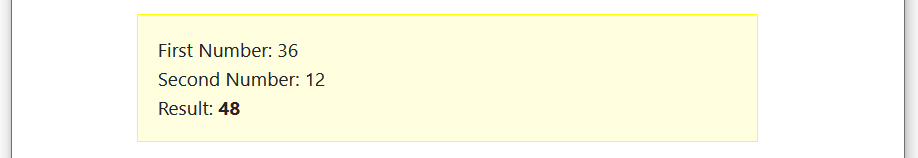Experiment with the file by changing the first and second number values, and by changing the arithmetic operator. You can close the exercise-3-2.html file when finished.

## Operator precedence

JavaScript follows the rules of arithmetic when working with numeric variables and numbers:

• Division first, followed by multiplication, then addition and finally subtraction.

For example, the following series of statements gives a result of 11 because JavaScript first multiplies 2 by 3 (resulting in 6) and then adds 5.

```let x = 5;
let y = 2;
let z = 3;
let answer = x + y * z;
```

You can force JavaScript to perform calculations in a particular order by using parentheses.

For example, the following calculation gives a result of 21. JavaScript first adds 5 and 2 (because they are within parentheses) and then multiplies that result by 3 to give 21.

```let x = 5;
let y = 2;
let z = 3;
let answer = (x + y) * z;
```

Ensure that you follow every opening bracket with a matching closing bracket. For example.

```let result = ((8 * distance) + tax) / (volume - 7)
```

In Exercise 3.3 you will use parentheses in a calculation that converts between temperature scales.

### Exercise 3.3: Using parentheses in calculations

exercise-3-3.html

In the empty <script></script> tag pair in the web page insert the declarations and assignments shown below.

```/* Declare variables */
let fahrenheit;
let celsius;
celsius = 220;
```

Next, insert the arithmetic statement that performs the temperature conversion calculation.

```/* Perform calculation */
fahrenheit = ((9/5) * celsius ) + 32;
```

Finally, insert two document.write() statements to display the calculation input and output on the web page.

```/* Display input and result */
document.write("Temperature (<i>Celsius<\/i>): "+celsius+"&deg;<br>");
document.write("Temperature (<i>Fahrenheit<\/i>): "+fahrenheit+"&deg;");
```

Your complete code should now look as follows.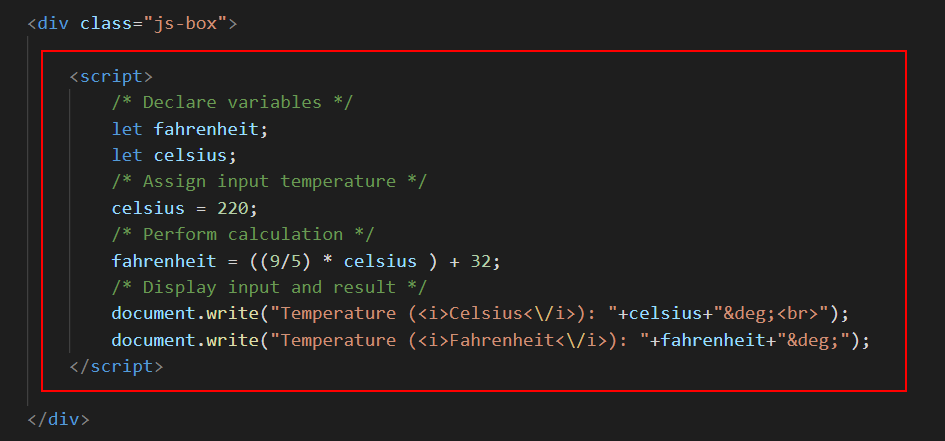Experiment with the file by changing the input (Fahrenheit) temperature value.

You can close the exercise-3-3.html file when finished.

## About the assignment (=) operator

Putting a value into a variable is known as assignment, and is achieved through the use of the assignment operator (=). For example:

```fruit = "apple";
```

This places the value of ‘apple’ inside the variable named ‘fruit'. Switching around this assignment statement results in the value of ‘fruit’ being placed inside the variable named ‘apple'.

```apple = "fruit";
```

In JavaScript the equals sign does not mean 'equal to' as it does in arithmetic or algebra. It means as follows:

‘Take whatever is on the right of the equals sign and place it in variable on the left hand side.'

JavaScript treats the left-hand side (LHS) as a variable, but the right-hand side (RHS) may be a value, another variable, or an object property. In the example below the RHS contains a value.

```country = "Bermuda";
```

Consider the next example. Here the RHS contains another variable. What this assignment does is copy whatever value is in the ‘cost’ variable to the ‘price’ variable.

```price = cost;
```

It's perfectly valid to place the same variable on both sides of the assignment operator. The following example puts the current content of the StreetAddress variable inside the StreetAddress variable. Nothing is changed as a result of this statement but no syntax rule is broken either.

```StreetAddress = StreetAddress;
```

Sometimes you may want to change a variable's value by a fixed amount. The following would make no sense if it where algebra.

```SalePrice = SalePrice + 20;
```

But in JavaScript it means ‘jack up the sale price by 20'. That is, insert in the sale price variable whatever is currently in that variable already plus 20.

JavaScript does not accept an assignment statement with a value on both sides. The LHS must always be a variable or an object property. Running the following statement generates an error in the web browser window.

```32 = 32;
```

Assignment statement

A JavaScript statement in which a variable or object property on the left of the equal sign (=) is given the value of whatever is on the right of the equals sign.

## Automatic variable retyping

JavaScript, as you have learnt, decides which type of variable you want to create by the way that you first assign a value to it. When you enclose the value within quotes, JavaScript makes the variable a string variable; when you omit the quotes, JavaScript makes it a numeric variable.

JavaScript can also change the type of a variable when you assign a value of a different type to that variable. It's called automatic type conversion. Consider the following two statements.

```let myvar = "12.34";
myvar = 12.34;
```

In the first statement JavaScript makes the variable named ‘myvar’ a string variable. In the second statement JavaScript changes the variable type to numeric because a number was assigned to it.

Automatic variable type conversion is a powerful feature of JavaScript, but its very power can result in scripting errors or in scripts that produce unexpected results. You need to be particularly careful with variables types when dealing with HTML forms that accept input from web page visitors. Because forms accept input as strings you need to convert such strings to numbers when you want to include them in arithmetic calculations.

### The typeof operator

When you are unsure about variable type at any some point in a script you can use the typeof operator to reveal the variable type. You insert the typeof operator directly before the variable. Here are three statements in which the typeof operator is used within a document.write() statement.

```document.write(typeof SalesPrice);
document.write(typeof x);
```

Exercise 3.4 takes you through the steps setting, changing and displaying a variable's type.

### Exercise 3.4: Investigating a variable’s type

Open the following file in VS Code and web browser:

exercise-3-4.html

In the empty <script></script> tag pair in the web page insert the following code.

```let firstVar;
document.write("<b>Variable Type:<\/b> " + typeof firstVar);
```

Save and reload the web page. In the browser window you can see that the variable type is 'undefined'. This is because you have only declared the variable but not assigned a value to it.Next, replace everything within the <script></script> tag pair with the following code.

```let firstVar;
document.write("<p><b>Variable Type:<\/b> " + typeof firstVar+"<\/p>");
document.write("<p><b>Variable Type:<\/b> " + typeof firstVar+"<\/p>");
document.write("<p><b>Variable Type:<\/b> " + typeof firstVar+"<\/p>");
document.write("<p><b>Variable Type:<\/b> " + typeof firstVar+"<\/p>");
```

Save and reload your web page. You can see the result of four document.write() statements, each one displayed on a separate line.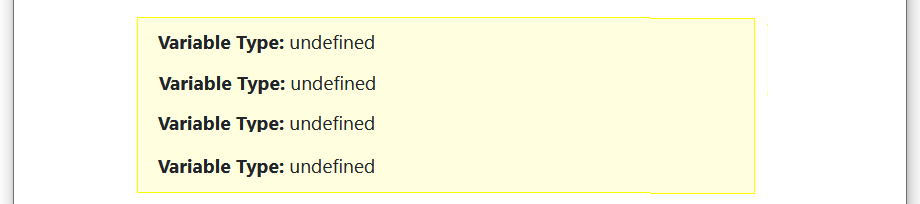Now, add three assignment statements to your block of code at the locations shown below.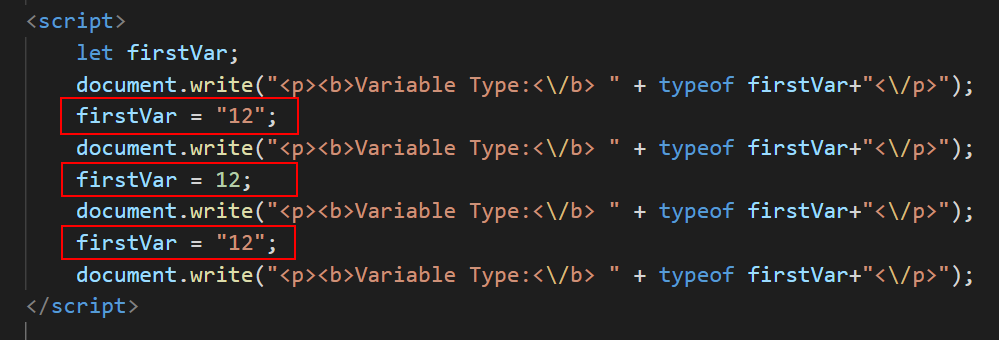Save and reload your web page. It should now look as shown below.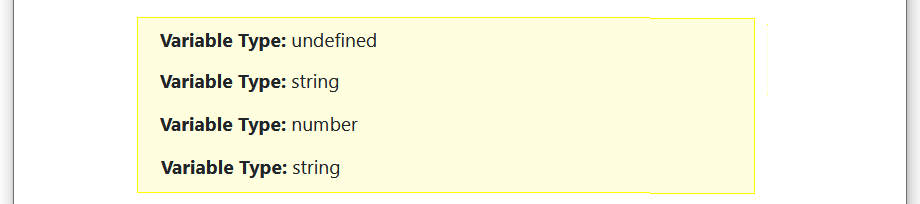You can see how the variable's type changed during the script: from undefined (no type), to numeric, then to string, and finally back to numeric again.

You can close the exercise-3-4.html file when finished.

1. Sign in to Github and click the name of the repository (‘repo’) that holds your web pages.
2. On the next GitHub screen displayed, click the Add file button near the right of the screen and then choose Upload files from the dropdown list.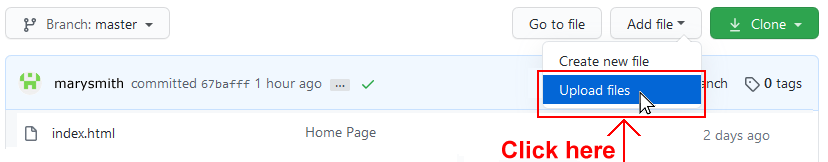3. In File Explorer (Windows 10) or Finder (Apple Mac), select your entire 📁 /javascript sub-folder, and drag-and-drop it to your repository on GitHub.4. Scroll down to the bottom of the GitHub screen, and accept or edit the short message (Add files via upload) in the Commit changes box.   Finally, click the green Commit changes button to upload your entire 📁 /javascript sub-folder and all the exercise files it contains.

It may take a few minutes for your uploaded files to appear on GitHub.

## Lesson summary

You can think of a variable as a container for a value that can change. Variable names must be unique, are case sensitive and cannot contain spaces.

A string variable can contain any keyboard characters, whether letters, numbers, punctuation symbols or white spaces, or any combination of these. A numeric variable stores a number, whether an integer (whole number) or a floating-point (decimal) number.

Values stored in numeric variables can be used in arithmetic calculations. You can store a number in a string variable, but it cannot be used in a calculation.

In JavaScript the first thing you do with a variable is declare it. The following code declares the variable named FirstName. The declaration begins with the word let.

```let FirstName;
```

JavaScript decides which type of variable you want to create by the way you assign a value to it. When you enclose the value within quotes JavaScript makes the variable a string variable. For example.

```StreetAddress = "42 Green Avenue";
```

When you omit the quotes JavaScript makes the variable a numeric variable. For example.

```OrderQty = 4;
```

You can declare a variable and assign a value to it in a single statement as follows.

```userName ="Sheila";
userID= 634634;
```

Putting a value into a variable is known as assignment, and is achieved through the use of the assignment operator (=). The left hand side must always be a variable or an object property. The right hand side can be a value, a variable or an object property.

JavaScript's automatic type conversion feature means that you can change the type of a variable by assigning a different type of value to it. In the first of the two statements below JavaScript makes the variable named ‘myvar’ a string variable. In the second statement JavaScript changes the variable type to numeric because a number is assigned to it.

```let myvar = "12.34";
myvar = 12.34;
```

You can use the typeof operator to reveal a variable's type. The following example shows the typeof operator used within a document.write() statement.

```document.write(typeof SalePrice);
```

You can use the addition (+), subtraction (-), multiplication (*) and division (/) operators to perform arithmetic with numeric variables.

JavaScript follows the rules of arithmetic when working with numeric variables and numbers: division first, followed by multiplication, then addition and finally subtraction. You can force JavaScript to perform calculations in a particular order by using parentheses as shown in the example below.

```let result = ((8 * distance) + tax) / (volume - 7)
```

Ensure that you follow every opening bracket with a matching closing bracket.